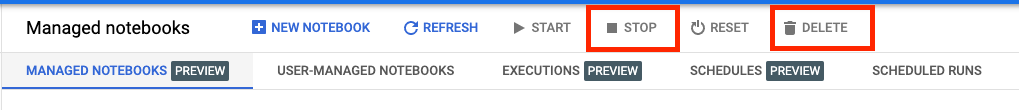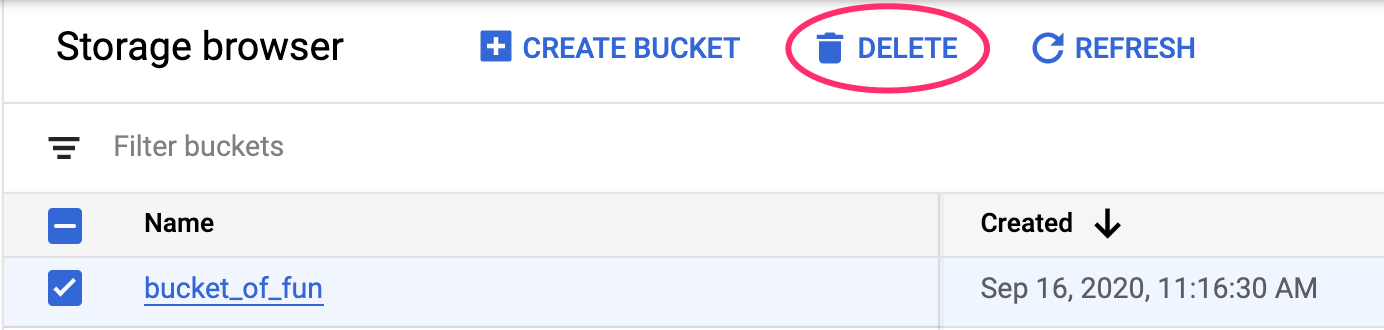# Prototype to Production: Hyperparameter tuning

## 1. Overview

In this lab, you'll use Vertex AI to run a hyperparameter tuning job on Vertex AI Training.

This lab is part of the Prototype to Production video series. Be sure to complete the previous lab before trying out this one. You can watch the accompanying video series to learn more:

.

## What you learn

You'll learn how to:

• Modify training application code for automated hyperparameter tuning
• Configure and launch a hyperparameter tuning job with the Vertex AI Python SDK

The total cost to run this lab on Google Cloud is about \$1.

## 2. Intro to Vertex AI

This lab uses the newest AI product offering available on Google Cloud. Vertex AI integrates the ML offerings across Google Cloud into a seamless development experience. Previously, models trained with AutoML and custom models were accessible via separate services. The new offering combines both into a single API, along with other new products. You can also migrate existing projects to Vertex AI.

Vertex AI includes many different products to support end-to-end ML workflows. This lab will focus on the products highlighted below: Training and Workbench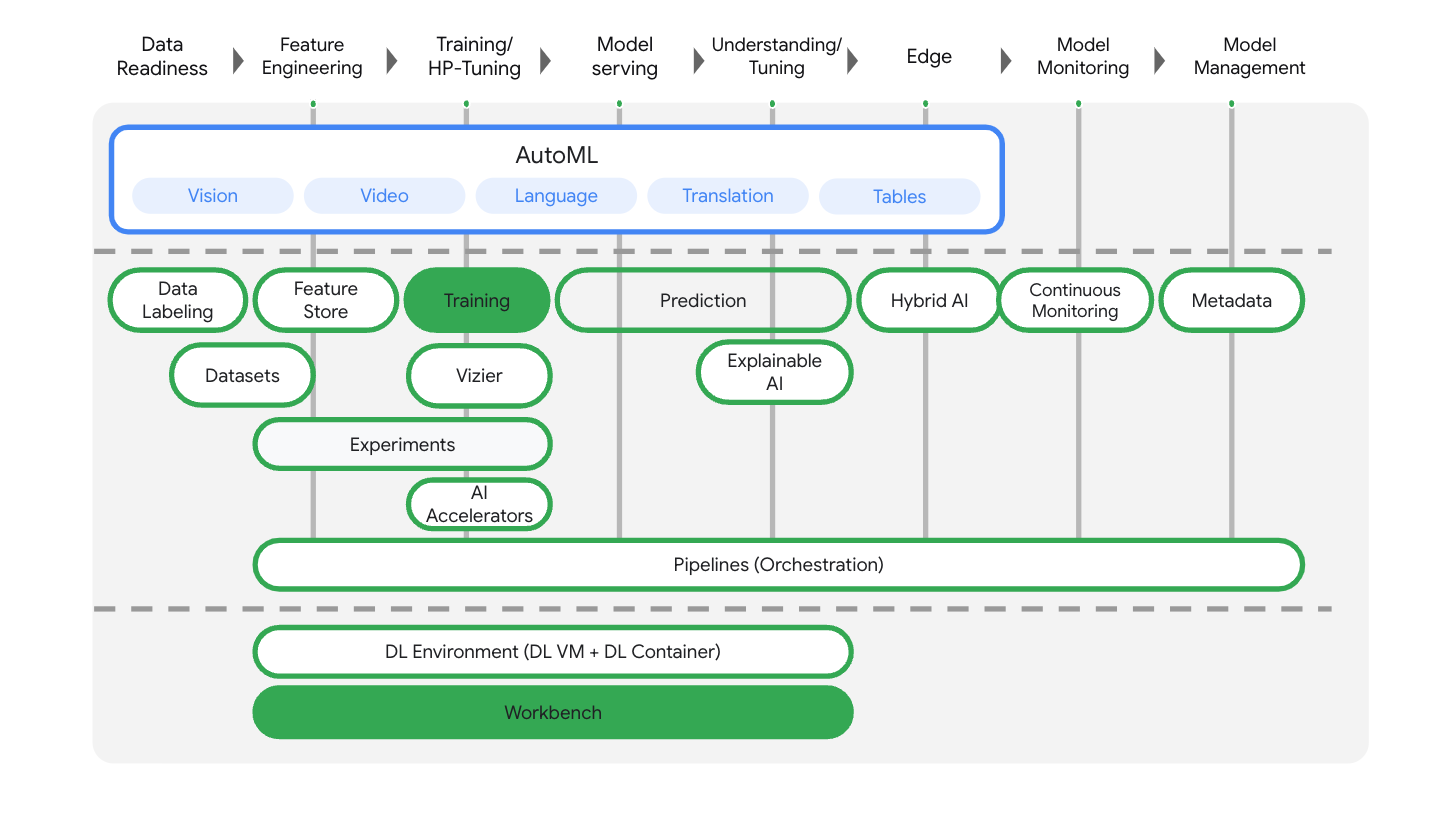## 3. Set up your environment

Complete the steps in the Training custom models with Vertex AI lab to set up your environment.

## 4. Containerize training application code

You'll submit this training job to Vertex AI by putting your training application code in a Docker container and pushing this container to Google Artifact Registry. Using this approach, you can train and tune a model built with any framework.

To start, from the Launcher menu of the Workbench notebook that you created in the previous labs, open a terminal window.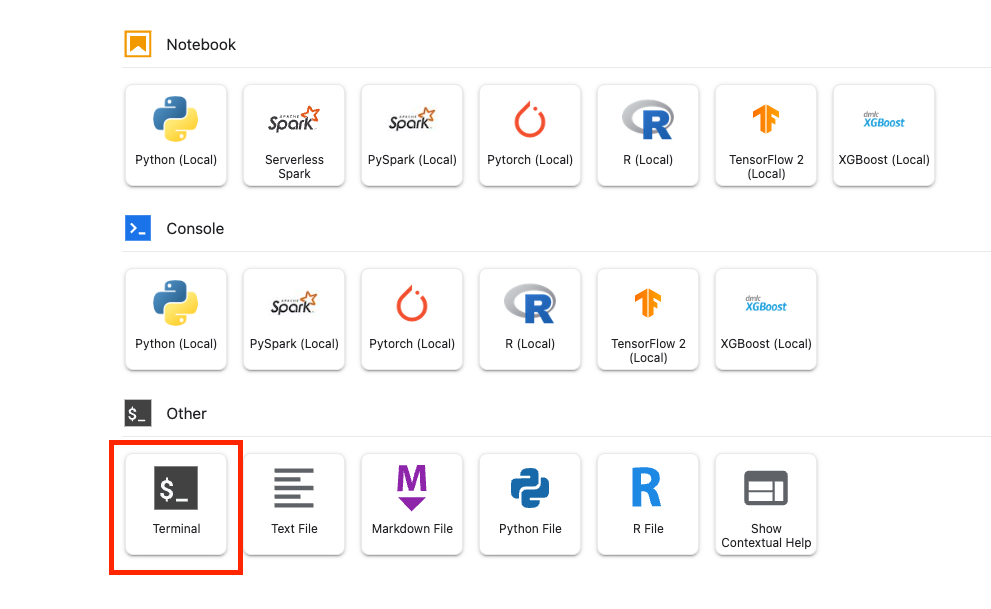## Step 1: Write training code

Create a new directory called `flowers-hptune` and cd into it:

``````mkdir flowers-hptune
cd flowers-hptune
``````

Run the following to create a directory for the training code and a Python file where you'll add the code below.

``````mkdir trainer
``````

You should now have the following in your `flowers-hptune/` directory:

``````+ trainer/
``````

Next, open the `task.py` file you just created and copy the code below.

You'll need to replace `{your-gcs-bucket}` in `BUCKET_ROOT` with the Cloud Storage bucket where you stored the flowers dataset in Lab 1.

``````import tensorflow as tf
import numpy as np
import os
import hypertune
import argparse

## Replace {your-gcs-bucket} !!
BUCKET_ROOT='/gcs/{your-gcs-bucket}'

# Define variables
NUM_CLASSES = 5
EPOCHS=10
BATCH_SIZE = 32

IMG_HEIGHT = 180
IMG_WIDTH = 180

DATA_DIR = f'{BUCKET_ROOT}/flower_photos'

def get_args():
'''Parses args. Must include all hyperparameters you want to tune.'''

parser = argparse.ArgumentParser()
'--learning_rate',
required=True,
type=float,
help='learning rate')
'--momentum',
required=True,
type=float,
help='SGD momentum value')
'--num_units',
required=True,
type=int,
help='number of units in last hidden layer')
args = parser.parse_args()
return args

def create_datasets(data_dir, batch_size):
'''Creates train and validation datasets.'''

train_dataset = tf.keras.utils.image_dataset_from_directory(
data_dir,
validation_split=0.2,
subset="training",
seed=123,
image_size=(IMG_HEIGHT, IMG_WIDTH),
batch_size=batch_size)

validation_dataset = tf.keras.utils.image_dataset_from_directory(
data_dir,
validation_split=0.2,
subset="validation",
seed=123,
image_size=(IMG_HEIGHT, IMG_WIDTH),
batch_size=batch_size)

train_dataset = train_dataset.cache().shuffle(1000).prefetch(buffer_size=tf.data.AUTOTUNE)
validation_dataset = validation_dataset.cache().prefetch(buffer_size=tf.data.AUTOTUNE)

return train_dataset, validation_dataset

def create_model(num_units, learning_rate, momentum):
'''Creates model.'''

model = tf.keras.Sequential([
tf.keras.layers.Resizing(IMG_HEIGHT, IMG_WIDTH),
tf.keras.layers.Rescaling(1./255, input_shape=(IMG_HEIGHT, IMG_WIDTH, 3)),
tf.keras.layers.MaxPooling2D(),
tf.keras.layers.MaxPooling2D(),
tf.keras.layers.MaxPooling2D(),
tf.keras.layers.Flatten(),
tf.keras.layers.Dense(num_units, activation='relu'),
tf.keras.layers.Dense(NUM_CLASSES, activation='softmax')
])

model.compile(optimizer=tf.keras.optimizers.SGD(learning_rate=learning_rate, momentum=momentum),
loss=tf.keras.losses.SparseCategoricalCrossentropy(),
metrics=['accuracy'])

return model

def main():
args = get_args()
train_dataset, validation_dataset = create_datasets(DATA_DIR, BATCH_SIZE)
model = create_model(args.num_units, args.learning_rate, args.momentum)
history = model.fit(train_dataset, validation_data=validation_dataset, epochs=EPOCHS)

# DEFINE METRIC
hp_metric = history.history['val_accuracy'][-1]

hpt = hypertune.HyperTune()
hpt.report_hyperparameter_tuning_metric(
hyperparameter_metric_tag='accuracy',
metric_value=hp_metric,
global_step=EPOCHS)

if __name__ == "__main__":
main()

``````

Before you build the container, let's take a deeper look at the code. There are a few components that are specific to using the hyperparameter tuning service.

1. The script imports the `hypertune` library.
2. The function `get_args()` defines a command-line argument for each hyperparameter you want to tune. In this example, the hyperparameters that will be tuned are the learning rate, the momentum value in the optimizer, and the number of units in the last hidden layer of the model, but feel free to experiment with others. The value passed in those arguments is then used to set the corresponding hyperparameter in the code.
3. At the end of the `main()` function, the `hypertune` library is used to define the metric you want to optimize. In TensorFlow, the keras `model.fit` method returns a `History` object. The `History.history` attribute is a record of training loss values and metrics values at successive epochs. If you pass validation data to `model.fit` the `History.history` attribute will include validation loss and metrics values as well. For example, if you trained a model for three epochs with validation data and provided `accuracy` as a metric, the `History.history` attribute would look similar to the following dictionary.
``````{
"accuracy": [
0.7795261740684509,
0.9471358060836792,
0.9870933294296265
],
"loss": [
0.6340447664260864,
0.16712145507335663,
0.04546636343002319
],
"val_accuracy": [
0.3795261740684509,
0.4471358060836792,
0.4870933294296265
],
"val_loss": [
2.044623374938965,
4.100203514099121,
3.0728273391723633
]
``````

If you want the hyperparameter tuning service to discover the values that maximize the model's validation accuracy, you define the metric as the last entry (or `NUM_EPOCS - 1`) of the `val_accuracy` list. Then, pass this metric to an instance of `HyperTune`. You can pick whatever string you like for the `hyperparameter_metric_tag`, but you'll need to use the string again later when you kick off the hyperparameter tuning job.

## Step 2: Create a Dockerfile

To containerize your code, you'll need to create a Dockerfile. In the Dockerfile you'll include all the commands needed to run the image. It'll install all the necessary libraries and set up the entry point for the training code.

From your Terminal, create an empty Dockerfile in the root of your `flowers-hptune` directory:

``````touch Dockerfile
``````

You should now have the following in your `flowers-hptune/` directory:

``````+ Dockerfile
+ trainer/
``````

Open the Dockerfile and copy the following into it. You'll notice that this is almost identical to the Dockerfile we used in the first lab, except now we're installing the cloudml-hypertune library.

``````FROM gcr.io/deeplearning-platform-release/tf2-gpu.2-8

WORKDIR /

# Installs hypertune library
RUN pip install cloudml-hypertune

# Copies the trainer code to the docker image.
COPY trainer /trainer

# Sets up the entry point to invoke the trainer.
``````

## Step 3: Build the container

From your Terminal, run the following to define an env variable for your project, making sure to replace `your-cloud-project` with the ID of your project:

``````PROJECT_ID='your-cloud-project'
``````

Define a repo in Artifact Registry. We'll use the repo we created in the first lab.

``````REPO_NAME='flower-app'
``````

Define a variable with the URI of your container image in Google Artifact Registry:

``````IMAGE_URI=us-central1-docker.pkg.dev/\$PROJECT_ID/\$REPO_NAME/flower_image_hptune:latest
``````

Configure docker

``````gcloud auth configure-docker \
us-central1-docker.pkg.dev
``````

Then, build the container by running the following from the root of your `flower-hptune` directory:

``````docker build ./ -t \$IMAGE_URI
``````

Lastly, push it to Artifact Registry:

``````docker push \$IMAGE_URI
``````

With the container pushed to Artifact Registry, you're now ready to kick off a the training job.

## 5. Run hyperparameter tuning job with the SDK

In this section, you'll learn how to configure and submit the hyperparameter tuning job by using the Vertex Python API.

From the Launcher, create a TensorFlow 2 notebook.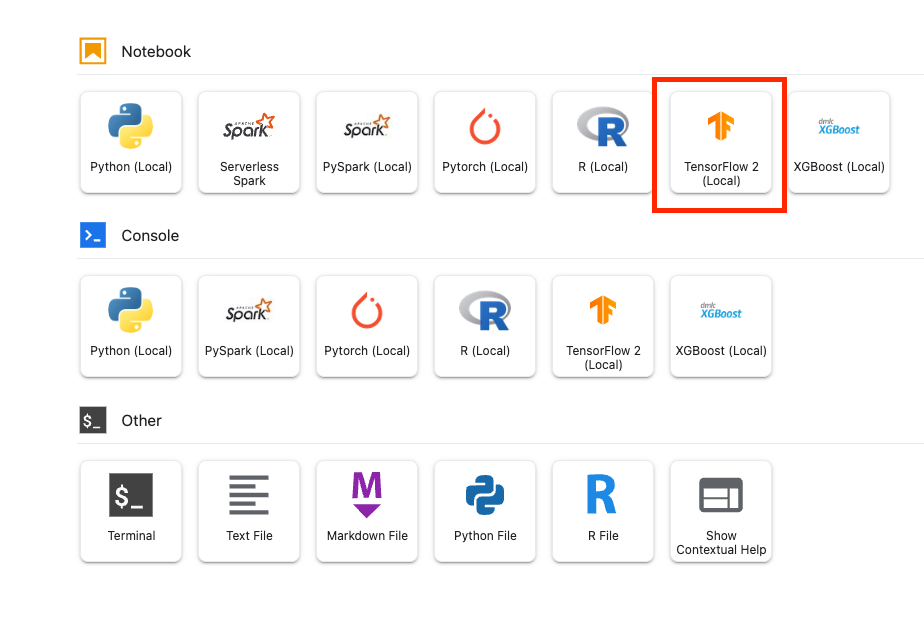Import the Vertex AI SDK.

``````from google.cloud import aiplatform
from google.cloud.aiplatform import hyperparameter_tuning as hpt
``````

To launch the hyperparameter tuning job, you need to first define the `worker_pool_specs`, which specifies the machine type and Docker image. The following spec defines one machine with two NVIDIA Tesla V100 GPUs.

You'll need to replace `{PROJECT_ID}` in the `image_uri` with your project.

``````# The spec of the worker pools including machine type and Docker image
# Be sure to replace PROJECT_ID in the `image_uri` with your project.

worker_pool_specs = [{
"machine_spec": {
"machine_type": "n1-standard-4",
"accelerator_type": "NVIDIA_TESLA_V100",
"accelerator_count": 1
},
"replica_count": 1,
"container_spec": {
"image_uri": "us-central1-docker.pkg.dev/{PROJECT_ID}/flower-app/flower_image_hptune:latest"
}
}]
``````

Next, define the `parameter_spec`, which is a dictionary specifying the parameters you want to optimize. The dictionary key is the string you assigned to the command line argument for each hyperparameter, and the dictionary value is the parameter specification.

For each hyperparameter, you need to define the Type as well as the bounds for the values that the tuning service will try. Hyperparameters can be of type Double, Integer, Categorical, or Discrete. If you select the type Double or Integer, you'll need to provide a minimum and maximum value. And if you select Categorical or Discrete you'll need to provide the values. For the Double and Integer types, you'll also need to provide the Scaling value. You can learn more about how to pick the best scale in this video.

``````# Dictionary representing parameters to optimize.
# The dictionary key is the parameter_id, which is passed into your training
# job as a command line argument,
# And the dictionary value is the parameter specification of the metric.
parameter_spec = {
"learning_rate": hpt.DoubleParameterSpec(min=0.001, max=1, scale="log"),
"momentum": hpt.DoubleParameterSpec(min=0, max=1, scale="linear"),
"num_units": hpt.DiscreteParameterSpec(values=[64, 128, 512], scale=None)
}
``````

The final spec to define is `metric_spec`, which is a dictionary representing the metric to optimize. The dictionary key is the `hyperparameter_metric_tag` that you set in your training application code, and the value is the optimization goal.

``````# Dictionary representing metric to optimize.
# The dictionary key is the metric_id, which is reported by your training job,
# And the dictionary value is the optimization goal of the metric.
metric_spec={'accuracy':'maximize'}
``````

Once the specs are defined, you'll create a CustomJob, which is the common spec that will be used to run your job on each of the hyperparameter tuning trials.

You'll need to replace `{YOUR_BUCKET}` with the bucket you created earlier.

``````# Replace YOUR_BUCKET
my_custom_job = aiplatform.CustomJob(display_name='flowers-hptune-job',
worker_pool_specs=worker_pool_specs,
staging_bucket='gs://{YOUR_BUCKET}')
``````

Then, create and run the `HyperparameterTuningJob`.

``````hp_job = aiplatform.HyperparameterTuningJob(
display_name='flowers-hptune-job',
custom_job=my_custom_job,
metric_spec=metric_spec,
parameter_spec=parameter_spec,
max_trial_count=15,
parallel_trial_count=3)

hp_job.run()
``````

There are a few arguments to note:

• max_trial_count: You'll need to put an upper bound on the number of trials the service will run. More trials generally leads to better results, but there will be a point of diminishing returns, after which additional trials have little or no effect on the metric you're trying to optimize. It is a best practice to start with a smaller number of trials and get a sense of how impactful your chosen hyperparameters are before scaling up.
• parallel_trial_count: If you use parallel trials, the service provisions multiple training processing clusters. Increasing the number of parallel trials reduces the amount of time the hyperparameter tuning job takes to run; however, it can reduce the effectiveness of the job overall. This is because the default tuning strategy uses results of previous trials to inform the assignment of values in subsequent trials.
• search_algorithm: You can set the search algorithm to grid, random, or default (None). The default option applies Bayesian optimization to search the space of possible hyperparameter values and is the recommended algorithm. You can learn more about this algorithm here.

In the console, you'll be able to see the progress of your job.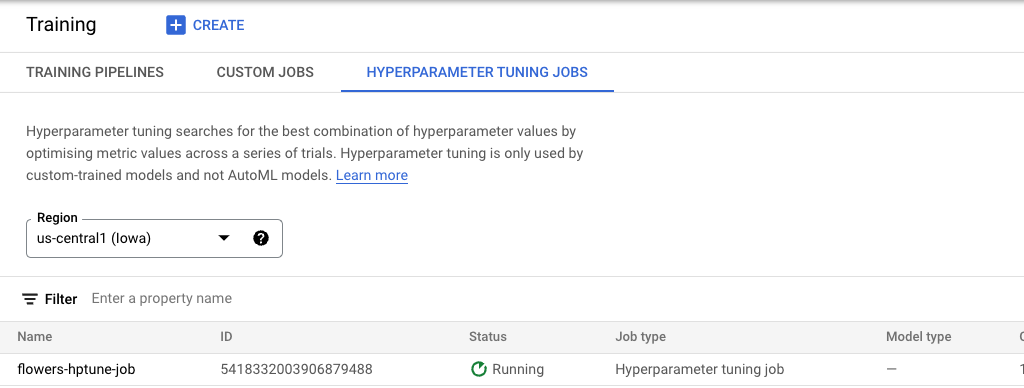And when it's finished, you can see the results of each trial and which set of values performed the best.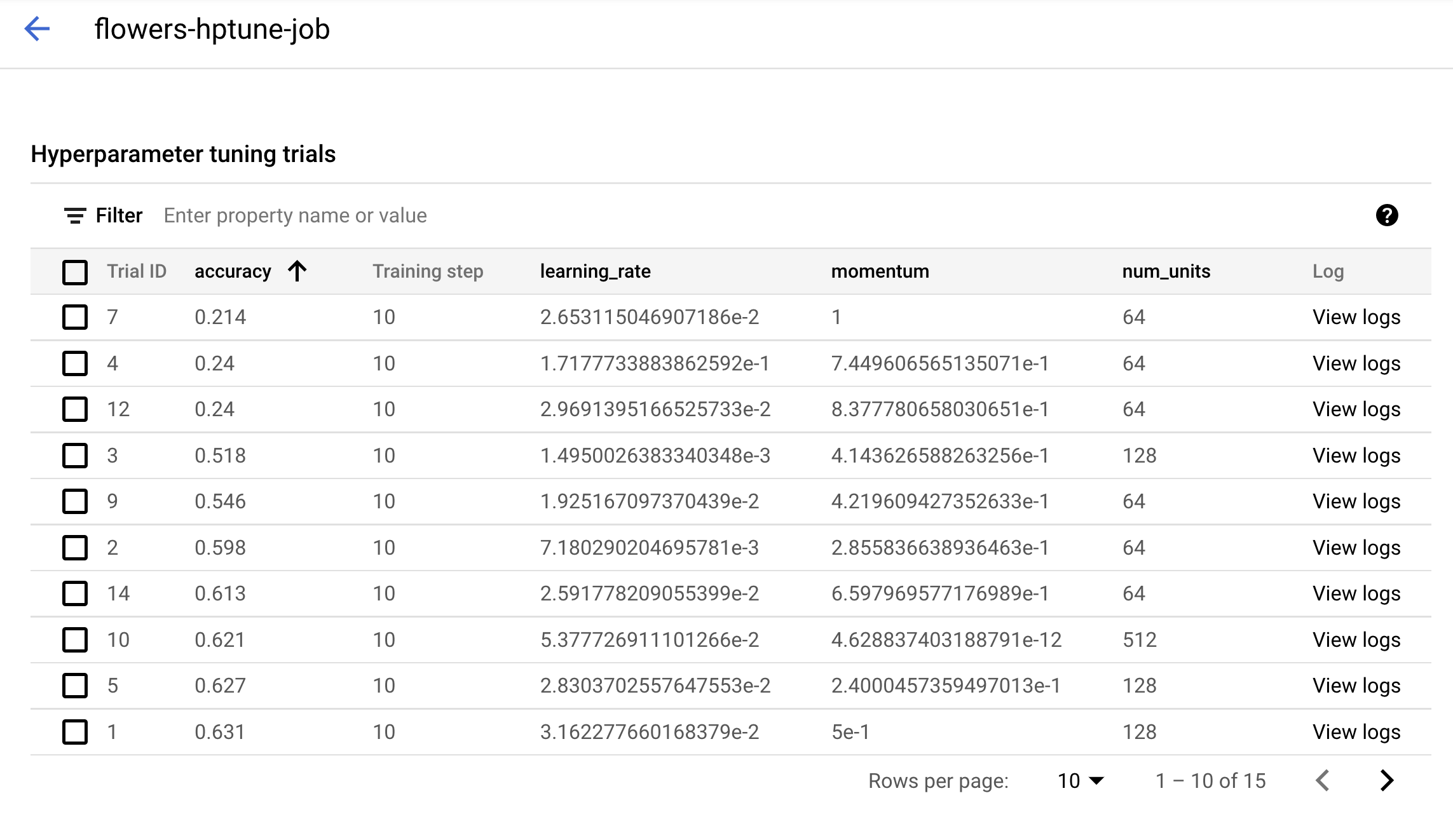🎉 Congratulations! 🎉

You've learned how to use Vertex AI to:

• Run an automated hyperparameter tuning job